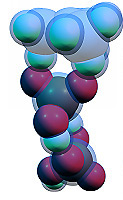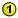## Console editor

This window allows various geometrical parameters to be changed (bond lengths, bond angles, dihedral angles) in the displayed molecule. To set new parameters in the structure, type their values in the textbox in the following format:

r(n1-n2)=value

r(n1-n2)=r(n3-n4)

r(n1-n2)=r(n3-n4)=value

a(n1-n2-n3)=value

a(n1-n2-n3)=a(n4-n5-n6)

a(n1-n2-n3)=a(n4-n5-n6)=value

d(n1-n2-n3-n4)=value

d(n1-n2-n3-n4)=d(n5-n6-n7-n8)

d(n1-n2-n3-n4)=d(n5-n6-n7-n8)=value

Here  n1,n2� are the numbers of atoms in the molecule, value - digital expression for the parameter or the expression of another parameter (for example, r(1-2)=r(1-3)). It is useful to press thebutton before using this window. Several parameters of equal value can be entered in one line, for example: a(2-1-3)=a(2-1-4)=a(3-1-4)=120). Here are some examples of using this utility:

r(1-2)=r(1-3)=r(1-4)=r(2-3)=r(2-4)=r(3-4)=3

The atoms 1,2,3,4 will form an equilateral tetrahedron with the edge length of 3 angstroms.

a(2-1-3)=a(2-1-4)=a(3-1-4)=30

r(1-2)=r(1-3)=r(1-4)

r(2-3)=2

The atoms 1,2,3,4 will form an isosceles pyramid with the apical angles of 30 degrees and the base edge's length of 2 angstroms.

Note: In some cases, it is necessary to set the initial positions of atoms close to the required geometry before using this editor.

BACK